## Myers' Diff Algorithm : The linear space refinement

Investigating Myers' diff algorithm: Part 2 of 2.
By Nicholas ButlerPublicly recommend on Google

## Introduction

This article is an examination of the linear space refinement, as described by Eugene W. Myers in his paper, "An O(ND) Difference Algorithm and Its Variations". It was published in the journal "Algorithmica" in November 1986. The paper is available as a PDF here[^].

This is the second in a two part article about Myers' diff algorithm and requires an understanding of the basic greedy algorithm described in part I here [^].

The application available in the downloads is a learning aid. It displays a graphical representation of the various stages of the algorithm. I suggest you use it while reading this article to help understand the algorithm. The graphs in this article are screenshots of the application.

## Reverse Algorithm

The basic algorithm can also work in reverse, that is working from ( N, M ) back to ( 0, 0 ). The only difference is that the moves begin at the deletion / insertion / match instead of ending at them. Here is the solution of the example, but working in reverse.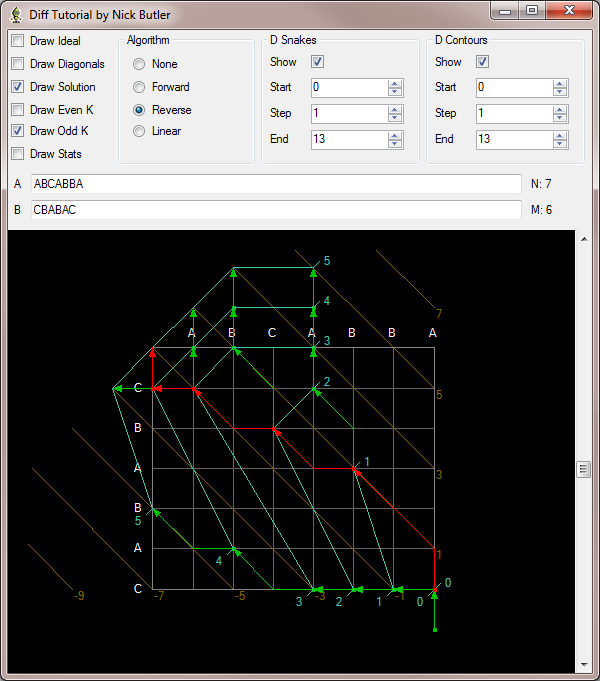Figure 1: Reverse solution

As you can see from this graph, the solution is different from the one generated by working forward, but has the same lengths of the LCS and SES. This is entirely correct, because in general there can be many equivalent solutions and the algorithm just chooses the first one it finds.

Delta: Because the lengths of the sequences A and B can be different, the k lines of the forward and reverse algorithms can be different. It is useful to isolate this difference as a variable `delta = N - M`. In the example, N = 7 and M = 6 which gives delta = 1. This is the offset from the forward k lines to the reverse ones. You can say that forward paths are centred around k = 0 and reverse paths are centred around k = delta.

## Middle Snake

### Overlap

You can run the forward and reverse algorithms at the same time for successive values of D. At some value of D, two snakes will overlap on some k line. The paper proves that one of these snakes is part of a solution. As it will be somewhere in the middle, it is called the middle snake.

The middle snake for the example is shown in pink in this graph: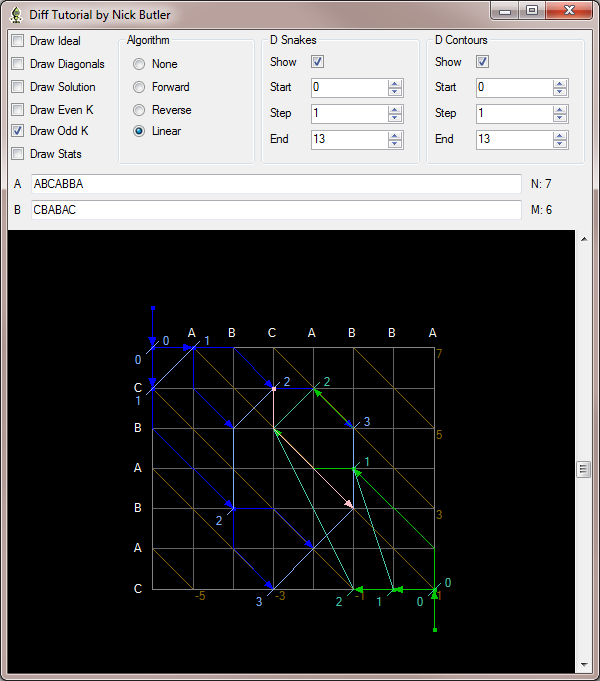Figure 2: Middle snake

This is useful because it divides the problem into two parts, which can then be solved separately and recursively.

This is linear in space because only the last V vectors must be stored, giving O( D ). For time, this linear algorithm is still O ( (N+M) D ).

It also helps that the middle snake must be found with a D which is half the D of the forward and reverse algorithms. This means that as D increases, the time required approaches half that of the basic algorithms.

Below is the pseudo-code we have so far:

```for d = 0 to ( N + M + 1 ) / 2
{
for k = -d to d step 2
{
calculate the furthest reaching forward and reverse paths
if there is an overlap, we have a middle snake
}
}```

### Odd and Even Deltas

Each difference - a horizontal deletion or a vertical insertion - is a move from one k line to its neighbour. As delta is the difference between the centres of the forward and reverse algorithms, we know which values of d we need to check for a middle snake.

For odd delta, we must look for overlap of forward paths with differences d and reverse paths with differences d - 1.

This graph shows that for delta = 3, the overlap occurs when the forward d is 2 and the reverse d is 1: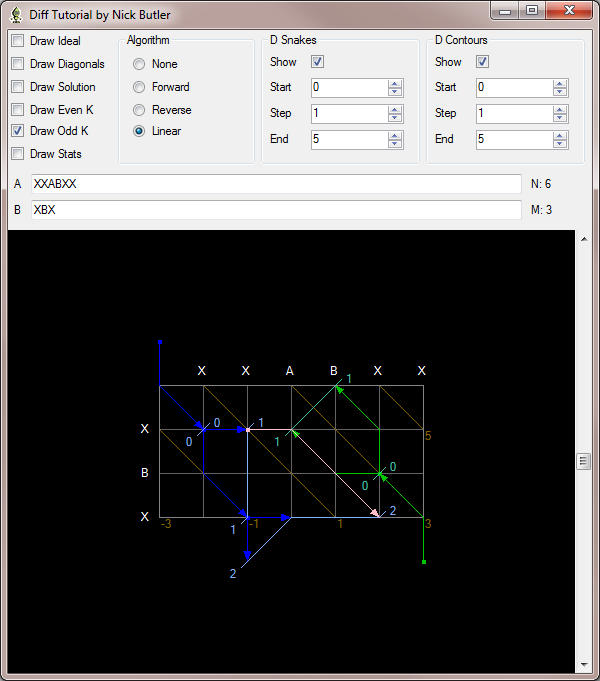Figure 3: Odd delta

Similarly for even delta, the overlap will be when both forward and reverse paths have the same number of differences.

The next graph shows that for delta = 2, the overlap occurs when the forward and reverse ds are both 2:Figure 4: Even delta

### Algorithm

So, here is the complete pseudo-code for finding the middle snake:

```delta = N - M
for d = 0 to ( N + M + 1 ) / 2
{
for k = -d to d step 2
{
calculate the furthest reaching forward path on line k
if delta is odd and ( k >= delta - ( d - 1 ) and k <= delta + ( d - 1 ) )
if overlap with reverse[ d - 1 ] on line k
=> found middle snake and SES of length 2D - 1
}

for k = -d to d step 2
{
calculate the furthest reaching reverse path on line k
if delta is even and ( k >= -d - delta and k <= d - delta )
if overlap with forward[ d ] on line k
=> found middle snake and SES of length 2D
}
}```

Note: The bounds checks of k are just to eliminate impossible overlaps.

## Recursive Solution

### Algorithm

We need to wrap the middle snake algorithm in a recursive method. Basically, we need to find a middle snake and then solve the rectangles that remain to the top left and bottom right. There are a couple of edge cases that I will explain in a moment. I have added the solution for the SES as the paper leaves this 'as an exercise' and just finds the LCS.

```Compare( A, N, B, M )
{
if ( M == 0 && N > 0 ) add N deletions to SES
if ( N == 0 && M > 0 ) add M insertions to SES
if ( N == 0 || M == 0 ) return

calculate middle snake

suppose it is from ( x, y ) to ( u, v ) with total differences D

if ( D > 1 )
{
Compare( A[ 1 .. x ], x, B[ 1 .. y ], y ) // top left

Compare( A[ u + 1 .. N ], N - u, B[ v + 1 .. M ], M - v ) // bottom right
}
else if ( D == 1 ) // must be forward snake
{
Add d = 0 diagonal to results
}
else if ( D == 0 ) // must be reverse snake
{
}
}```

I hope the general case is fairly clear. I will now explore the two edge cases.

### Edge case: D == 0

If the middle snake algorithm finds a solution with D = 0, then the two sequences are identical. This means that delta is zero, which is even. So the middle snake is a reverse snake which is just matches ( diagonals ). So all we have to do is add this snake to the results.

The graph below shows the general form of this edge case: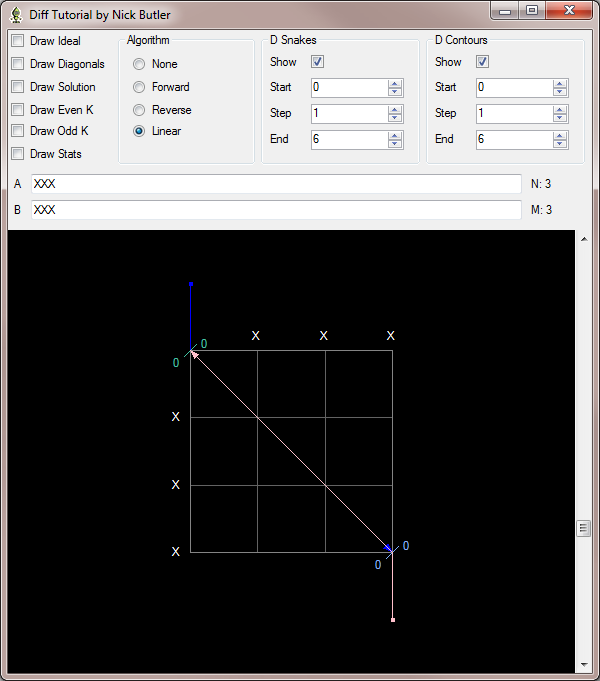Figure 5: Solution with D = 0

### Edge case: D == 1

If the middle snake algorithm finds a solution with D = 1, then there is either exactly one insertion or deletion. This means delta is 1 or -1 which are odd and so the middle snake is a forward snake.

We can complete the solution for this case by calculating the d = 0 diagonal and adding this, along with the middle snake, to the results.

The graph below shows the general form of this edge case: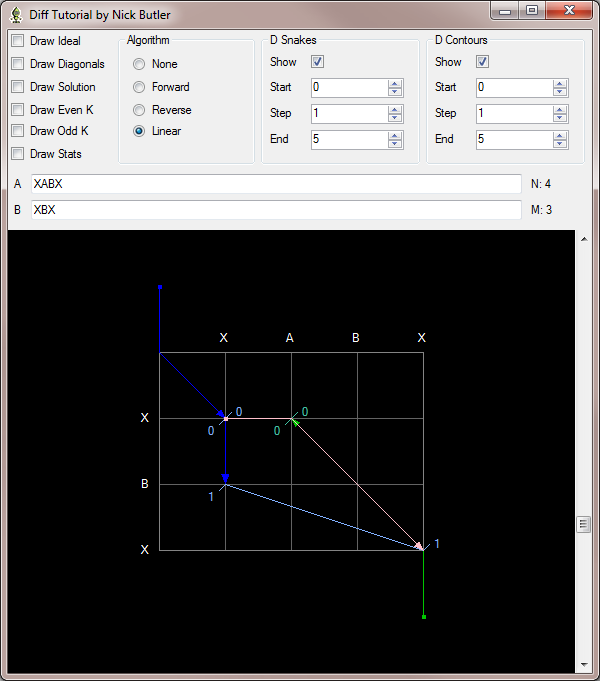Figure 6: Solution with D = 1

## Points of Interest

The middle snake algorithm is a work of art. It also allows for the recursive solution described and makes the algorithm embarrassingly parallel. I leave that implementation as an exercise.

There are other optimizations readily apparent, that trade space, which is cheaper in modern machines, for gains in time. However, this algorithm is such a good balance that it is still used by the *nix `diff` program, over 20 years after it was published.

## History

• 19th September, 2009: First edition